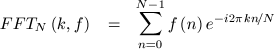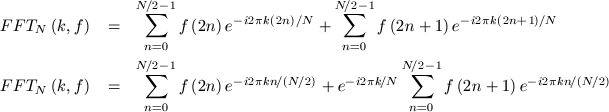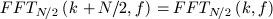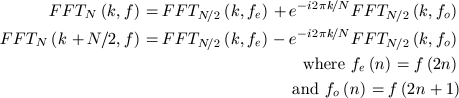If you want to see more math behind a Fourier Transform, read my DFT notes.

# Fast Fourier Transform

The DFT is a slow algorithm, over time much faster alternatives have been developed. One of the most popular is the Radix-2 Fast Fourier Transform which was developed in 1965 by Tukey and Cooley. It comes in two flavours - decimation in frequency, and decimation in time. I'll only deal with the latter.

The basic premise behind the Radix-2 Decimation in Time algorithm is that if we define an N-element FFT as:Then we can express one FFT of N inputs as two FFTs of N/2 inputs:The sub-transforms will only give us values for k between zero and N/2. The other half of the values are found by using the periodicity of the Fourier Transform:It looks a little neater if we split f into even and odd elements:We can express each one of those N/2-input FFTs as a sum of two N/4-input FFTs and so forth. As long as N is a power of two, we can keep splitting the FFT down until we get to a whole heap of 1-element transforms.

[fft1.c] My first attempt at implementing this was much faster than the DFT but very slow for an FFT because it was recursive and allocated memory dynamically to split f into evens and odds. (Because I wanted to see the math work before I launched into an even slightly complicated implementation)

[fft2.c] My second attempt - I sort the inputs into a bit-reversed order and then use a recursive, in-place FFT. No malloc abuse happens.

[fft3.c] My third attempt - eliminated recursion. There's still plenty of space for optimisation (such as taking advantage of trivial twiddle factors in the first few passes) but this is where I'm calling it quits.

# Faster Fourier Transforms

If you're looking for a really fast FFT implementation, try one of these:

# Fourier's Last Transform

rp: The process that transformed Fourier back to the elements of which he was composed.

Graphs by gnuplot.
If you spot any mathematical inaccuracies, give me a kick.

[home]
copyright © 2004 Emil Mikulic
First try: 2001/09/25
$Date: 2004/10/09 08:53:25$